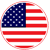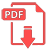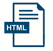# Home | Mathematics | Pre-Algebra

## Operations with Polynomials

Polynomials can be added or subtracted following a very simple rule: only coefficients of like terms can be added or subtracted. Like or similar terms are defined as those having the same variables raised to the same power.

For example, the terms 3x2, -5x2 are similar but the terms 3x2, -5y2 are not similar because they don't have the same variable, regardless of them having the same power.

Two terms are multiplied (assuming they have the same variable) by multiplying the coefficients and in a separate process, the variables are multiplied following the properties of the exponentials:

xm + xn = xm+nEnglish